# for loop in Python

Let's first see the syntax of the "for" loop. And then under the "Python for Loop Example" section, one by one, all the above versions of "for" get described with example programs.

## Python for Loop Syntax

The syntax to use a "for" loop in Python is as follows:

```for loop_variable in sequence:
statement_1
statement_2
statement_3
.
.
.
statement_n```

Or,

```for loop_variable in sequence:
body of the loop```

## Python for Loop Example

This section is designed to describe each version of the "for" loop using example programs.

### Iterating a string using a "for" loop

The for loop can be used to iterate all characters of a string, one by one, as shown in the program given below:

```string = "codescracker"
for c in string:
print(c)```

The output produced by the above program is shown in the snapshot given below: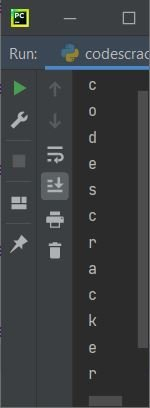The above program can also be written as:

```for c in "codescracker":
print(c)```

The dry run of the above program goes like this:

• At the first iteration, the first character of the string "codescracker," which is "c," gets initialized to the loop variable, which is "c."
• So using the print() function, the value of c, that is, "c," gets printed.
• At the second iteration, the second character of string "codescracker," which is "o," gets initialized to the loop variable, which is "c."
• Again, using print(), the new value of c, which is "o," gets printed.
• This process continues until the last character of the string "codescracker,"  which is "r."
• In this way, all the characters of a string get printed one by one, as shown in the snapshot given above.

That is, whatever you provide after

`for c in `

gets treated as a sequence. Because the string "codescracker" appears in the preceding program. Therefore, the string gets treated as a sequence, which is the sequence of characters. For example, if the preceding program is modified with the following program:

```for c in "codescracker", 2, True, 'c', 3.4:
print(c)```

Then, this time:

`"codescracker", 2, True, 'c', 3.4`

gets treated as a sequence. Therefore, one by one, all five elements get printed one by one, as shown in the snapshot given below: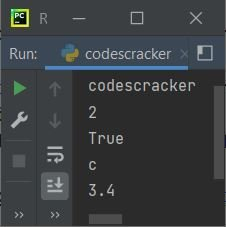Note that anything that is iterable in Python can be used as a sequence in a for loop. Since the "int" object is not iterable. As a result, the following program is required:

```for c in 1234:
print(c)```

produces an error like shown in the snapshot given below:### Iterating a list using a "for" loop

The list can also be iterated using a for loop, as shown in the program given below:

```mylist = [1, 2, 3, 4, 5]
for i in mylist:
print(i)```

The output produced by the above program is: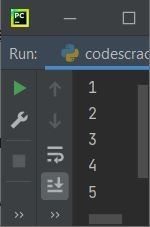The above program can also be written as:

```for i in [1, 2, 3, 4, 5]:
print(i)```

Or

```for i in 1, 2, 3, 4, 5:
print(i)```

The above program's dry run proceeds in the same manner as the previous program's dry run. That is, all five elements get initialized one by one in i (the loop variable of the above program). And the value of i gets printed as shown in the output given above.

Another program that uses a "for" loop to iterate through a list is as follows:

```mylist = ['P', 'Y', 'T', 'H', 'O', 'N']
for i in mylist:
print(i)```

Now the output produced by the above program is:

```P
Y
T
H
O
N```

Another program that uses a "for" loop to iterate through a list of words is:

```mylist = ['python', 'on', 'codes', 'cracker']
for i in mylist:
print(i)```

The snapshot given below shows the output produced by the above program: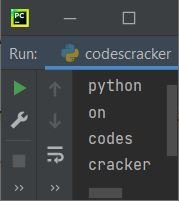This time, the list contains four words, and the list gets iterated by elements without caring what the element is, that is, whether it is a number, character, word, etc. The elements get iterated one by one.

### Iterating "for" the loop using the range() function

As far as I've seen while programming in Python for the last 5–6 years. Most of the time, the for loop comes with the range() function. That is, most of the time, we need  range() in a "for" loop. Here is the simplest Python code for a "for" loop with range().

```for i in range(10):
print(i)```

The snapshot given below shows the sample output produced by the above program that uses the range() function in a "for" loop in Python: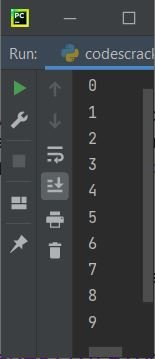In the above program, the range (10) generates a number starting with 0, increments by 1, and stops at 9 (one less than the value 10). That is, 0, 1, 2, 3, 4, 5, 6, 7, 8, and 9 are the 10 numbers that get generated by range(). And all the numbers get printed one by one, in the same way as in previous programs.

### "for" loop with range(), started by the specified value

If you provide two parameters to range(), then the iteration starts with the value of the first parameter. Let's create another version of the program that uses for loops with range(). This time, I've provided two parameters to range():

```for val in range(10, 20):
print(val)```

Now the output produced by the above program is: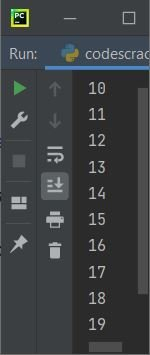Note: All the details about the range() function in Python are provided in a separate tutorial.

### "for" loop with range(), started and incremented by specified values

To increase the value of a loop variable by a specified value, we need to provide three parameters to range(). Because if you provide two parameters, the first parameter indicates where the iteration starts, and the second parameter indicates where the iteration stops. But by providing the third parameter, you indicate that you want to increment the loop variable by the value of the third parameter. Let's take a look at the program given below:

```for val in range(2, 22, 2):
print(val)```

The loop iteration starts with 2 and continues by incrementing 2 each time, stopping at 20 or 2 less before 22 (the second parameter), as shown in the output given below: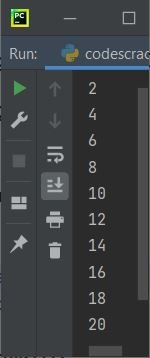### Iterating "for" loops in reverse or backward using range()

To iterate the for loop backwards or in reverse, we must pass three parameters to the range() function. The first parameter is the value, where the iteration starts. The second parameter is the value, where the iteration stops. And the third parameter's value must be in minus form to decrement the loop variable at each iteration. If -1 is provided, then the loop variable gets decremented by 1 each time.

```for val in range(10, 0, -1):
print(val)```

That is, the first parameter 10 refers to the number at which the iteration begins, the second parameter 0 refers to the end, but not with 0, because the iteration stops one before the number, i.e. 1, and the third parameter is provided with minus 1 (-1), allowing the iteration to be performed in reverse.

The output produced by the above program is shown in the snapshot given below: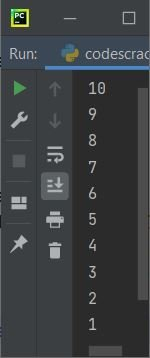Note: The third parameter is used to increment or decrement the loop variable. If the third parameter value is in positive form, then the loop variable gets incremented by the value. Otherwise, if a minus sign is given to the third parameter's value, then the loop variable's value gets decremented by that value each time.

Here is another program that prints the value in reverse, decremented by 2 each time:

```for val in range(10, 0, -2):
print(val)```

The output produced by the above program is:

```10
8
6
4
2```

### "for" loop with an else clause

The "else" used with the "for" loop is used to execute the statement when the execution of the "for" loop ends.

```for i in range(5):
print(i)
else:
print("Loop Execution finished!")```

The output produced by the above program is: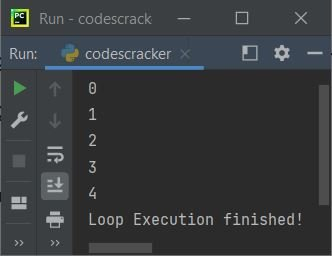### "break" statement in the "for" loop

The break statement or keyword inside a for loop is typically used to exit the loop without completing the loop's remaining iterations.

```mylist = [1, 2, 3, 4, 5, 6, 7, 8]
for i in mylist:
if i==6:
break
print(i)```

From the above program, the following block of code follows:

```if i==6:
break```

is used to exit from the loop when the value of i becomes equal to 6. The output produced by the above program will be:

```1
2
3
4
5```

### "continue" statement in the "for" loop

The "continue" statement inside the "for" loop is generally used to force the program flow to start with a new iteration without executing the remaining statement(s) after the "continue" keyword or statement, inside the same loop.

```mylist = [1, 2, 3, 4, 5, 6, 7, 8]
for i in mylist:
if i==6:
continue
print(i)```

In the above program, when the condition i==6 evaluates to true, program flow goes inside the if block. And the "continue" keyword gets executed. That forces the program flow to continue with a new iteration without executing the statement after the "continue" keyword in the same loop. That is, the statement:

`print(i)`

does not get executed when the value of i is 6. The output produced by the above program is shown in the snapshot given below:As you can see, 6 is not available on the output screen.

### "pass" statement in the "for" loop

The "pass" does nothing. Therefore, it is used where the syntax of the program needs statement(s), but we do not need to put or execute any statement. Let's take a look at the program given below that uses the pass statement inside the for loop in Python:

```print("Before \"for\" loop.")

for i in range(5):
pass

print("After \"for\" loop.")```

The output should be:

```Before "for" loop.
After "for" loop.```

Here is another program that uses pass inside the for loop in Python:

```print("Before \"for\" loop.")

for i in range(10):
if i==5 or i==6:
pass
else:
print(i)

print("After \"for\" loop.")```

This time, the output should be: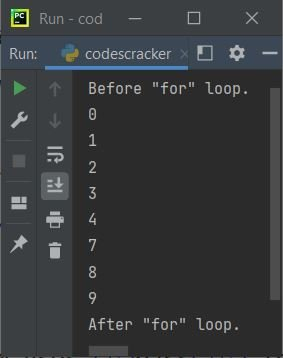That is, when the value of i becomes equal to 5 or 6, then the condition evaluates to be true, and the program flow goes inside the "if" block of "for." There, the "pass" just passes the program flow for the next iteration without doing anything.

## Nested "for" loop in Python

A loop in Python can also be nested inside another. For example, the program given below nests a for loop inside another:

```for i in range(5):
for j in range(i+1):
print("*", end=" ")
print()```

The output produced by the above program on a nested for loop is: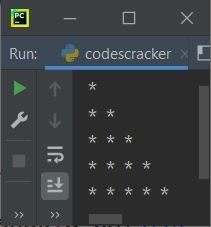Here is the brief dry run of the above program:

• At the first iteration, 0 gets initialized to i. Because 0 is less than 5, the program flow enters the loop with i = 0.
• Inside the loop, there is another for loop.
• Therefore, at the first iteration of the inner for loop, 0 gets initialized to j. Since 0 is less than i+1, 0+1, or 1, therefore, program flow goes inside the inner loop.
• Inside the second loop, a star (*) with a space gets printed on the output.
• The end is used to change the default behavior of print(). That is, instead of inserting a new line after each print(), The end of the above program forces print() to insert a space.
• Now the value of j gets incremented by 1. So j=1. But the value of j, that is, 1, is not less than i+1, or 1. Therefore, program flow does not go inside this loop.
• So the print() after the second for loop gets executed. That inserts a newline on the output screen.
• The value of i is now increased.So i=1. And again the value of i, that is, 1, is less than 5, therefore the program flow again goes inside the loop.
• inside the loop, since there is another for loop. So the execution of that second for loop again begins. with i = 1 this time. That is, initially j=0, which is less than i+1 or 1+1 or 2, therefore program flow goes inside the second for loop. So a * with a space gets printed.
• Now j = 1, which is again less than i + 1 or 2. Therefore, another * with a space gets printed.
• But at the third iteration, j=2 is not less than 2. Therefore, for now, the execution stops.
• And when print() gets executed, that inserts a newline.
• Now the value of i again gets incremented by 1. That is, i now equals 2. Since 2 is again less than 5, the program flow again goes inside the loop.
• This process continues until the condition is evaluated as false.

#### More Examples on for Loop

Python Online Test

« Previous Tutorial Next Tutorial »